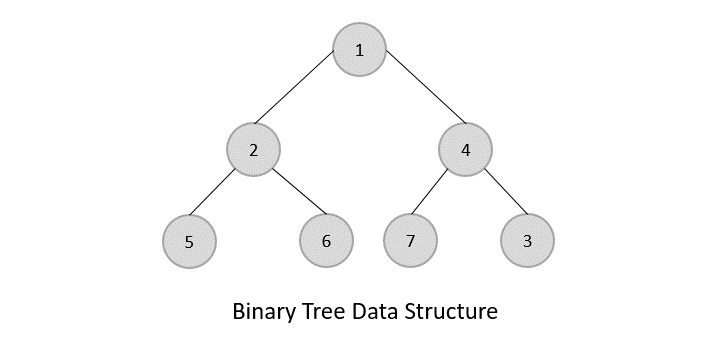# Data Structure and Algorithms - Tree

Advertisements

Tree represents the nodes connected by edges. We will discuss binary tree or binary search tree specifically.

Binary Tree is a special datastructure used for data storage purposes. A binary tree has a special condition that each node can have a maximum of two children. A binary tree has the benefits of both an ordered array and a linked list as search is as quick as in a sorted array and insertion or deletion operation are as fast as in linked list.## Important Terms

Following are the important terms with respect to tree.

• Path − Path refers to the sequence of nodes along the edges of a tree.

• Root − The node at the top of the tree is called root. There is only one root per tree and one path from the root node to any node.

• Parent − Any node except the root node has one edge upward to a node called parent.

• Child − The node below a given node connected by its edge downward is called its child node.

• Leaf − The node which does not have any child node is called the leaf node.

• Subtree − Subtree represents the descendants of a node.

• Visiting − Visiting refers to checking the value of a node when control is on the node.

• Traversing − Traversing means passing through nodes in a specific order.

• Levels − Level of a node represents the generation of a node. If the root node is at level 0, then its next child node is at level 1, its grandchild is at level 2, and so on.

• keys − Key represents a value of a node based on which a search operation is to be carried out for a node.

## Binary Search Tree Representation

Binary Search tree exhibits a special behavior. A node's left child must have a value less than its parent's value and the node's right child must have a value greater than its parent value.We're going to implement tree using node object and connecting them through references.

## Tree Node

The code to write a tree node would be similar to what is given below. It has a data part and references to its left and right child nodes.

```struct node {
int data;
struct node *leftChild;
struct node *rightChild;
};
```

In a tree, all nodes share common construct.

## BST Basic Operations

The basic operations that can be performed on a binary search tree data structure, are the following −

• Insert − Inserts an element in a tree/create a tree.

• Search − Searches an element in a tree.

• Preorder Traversal − Traverses a tree in a pre-order manner.

• Inorder Traversal − Traverses a tree in an in-order manner.

• Postorder Traversal − Traverses a tree in a post-order manner.

We shall learn creating (inserting into) a tree structure and searching a data item in a tree in this chapter. We shall learn about tree traversing methods in the coming chapter.

## Insert Operation

The very first insertion creates the tree. Afterwards, whenever an element is to be inserted, first locate its proper location. Start searching from the root node, then if the data is less than the key value, search for the empty location in the left subtree and insert the data. Otherwise, search for the empty location in the right subtree and insert the data.

### Algorithm

```If root is NULL
then create root node
return

If root exists then
compare the data with node.data

while until insertion position is located

If data is greater than node.data
goto right subtree
else
goto left subtree

endwhile

insert data

end If
```

### Implementation

The implementation of insert function should look like this −

```void insert(int data) {
struct node *tempNode = (struct node*) malloc(sizeof(struct node));
struct node *current;
struct node *parent;

tempNode->data = data;
tempNode->leftChild = NULL;
tempNode->rightChild = NULL;

//if tree is empty, create root node
if(root == NULL) {
root = tempNode;
} else {
current = root;
parent  = NULL;

while(1) {
parent = current;

//go to left of the tree
if(data < parent->data) {
current = current->leftChild;

//insert to the left
if(current == NULL) {
parent->leftChild = tempNode;
return;
}
}

//go to right of the tree
else {
current = current->rightChild;

//insert to the right
if(current == NULL) {
parent->rightChild = tempNode;
return;
}
}
}
}
}
```

## Search Operation

Whenever an element is to be searched, start searching from the root node, then if the data is less than the key value, search for the element in the left subtree. Otherwise, search for the element in the right subtree. Follow the same algorithm for each node.

### Algorithm

```If root.data is equal to search.data
return root
else
while data not found

If data is greater than node.data
goto right subtree
else
goto left subtree

If data found
return node
endwhile

return data not found

end if
```

The implementation of this algorithm should look like this.

```struct node* search(int data) {
struct node *current = root;
printf("Visiting elements: ");

while(current->data != data) {
if(current != NULL)
printf("%d ",current->data);

//go to left tree

if(current->data > data) {
current = current->leftChild;
}
//else go to right tree
else {
current = current->rightChild;
}

//not found
if(current == NULL) {
return NULL;
}

return current;
}
}
```

To know about the implementation of binary search tree data structure, please click here.

Advertisements